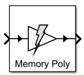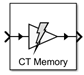# Power Amplifier

Model narrowband power amplifier with memory

Since R2022a

•Libraries:
RF Blockset / Idealized Baseband

## Description

The Power Amplifier block models a two-port power amplifier using a memory polynomial expression derived from the Volterra series. The Volterra series models the nonlinear relationship between input and output signals. This block includes memory effects: the output response depends on the current input signal and the input signal at previous times. Use this block when transmitting narrowband signals into your RF system.

The Power Amplifier block mask icons are dynamic and show the model specified in the Model Type parameter.

Model Type: `Memory polynomial`Model Type: `Cross-Term Memory`## Ports

### Input

expand all

Time-dependent input signal, specified as a real scalar, real column, complex scalar, or complex column. A column represents consecutive points in time.

Data Types: `double` | `single`

### Output

expand all

Time-dependent output signal, returned as a complex scalar or complex column. The output time-dependent signal is equal in size to the input time dependent signal.

Data Types: `double` | `single`

## Parameters

expand all

Use either the `Memory polynomial` model or the `Cross-Term Memory` model to design the power amplifier. The following table summarizes the characteristics of the two models.

ModelCharacterization DataType of CoefficientsIn-Band Spectral RegrowthOut-of-Band Harmonic Generation
`Memory polynomial` (default)Bandpass (I,Q)ComplexYesNo
`Cross-Term Memory`Bandpass (I,Q)ComplexYesNo

Coefficient matrix, specified as a complex matrix for the ```Memory polynomial``` and `Cross-Term Memory` models.

For the `Memory polynomial` and ```Cross-Term Memory``` models, you can identify the complex coefficient matrix based on the measured complex (I,Q) output-vs.-input amplifier characteristic. As an example, see the helper function in Coefficient Matrix Computation .

The size of the matrix depends on the number of delays and the degree of the system nonlinearity.

• For the `Memory polynomial` model, the matrix has dimensions .

• For the `Cross-Term Memory` model, the matrix has dimensions .

Sample time of the input-output data that the block uses to construct the coefficient matrix, specified in seconds.

The Time interval of measured PA data (s) parameter has been renamed to Measured interval of PA data (s). (since R2023b)

Type of simulation to run, specified as one of the following:

• `Code generation` — Simulate model using generated C code. The first time you run a simulation, Simulink® generates C code for the block. The C code is reused for subsequent simulations, as long as the model does not change. This option requires additional startup time, but the speed of the subsequent simulations is faster than `Interpreted execution`.

• `Interpreted execution` — Simulate model using the MATLAB® interpreter. This option shortens startup time speed, but the speed of the subsequent simulations is slower than ```Code generation```. In this mode, you can debug the source code of the block.

## Algorithms

expand all

 Morgan, Dennis R., Zhengxiang Ma, Jaehyeong Kim, Michael G. Zierdt, and John Pastalan. "A Generalized Memory Polynomial Model for Digital Predistortion of Power Amplifiers." IEEE Transactions on Signal Processing. Vol. 54, No. 10, October 2006, pp. 3852–3860.

 Gan, Li, and Emad Abd-Elrady. "Digital Predistortion of Memory Polynomial Systems using Direct and Indirect Learning Architectures". Proceedings of the Eleventh IASTED International Conference on Signal and Image Processing (SIP) (F. Cruz-Roldán and N. B. Smith, eds.), No. 654-802. Calgary, AB: ACTA Press, 2009.